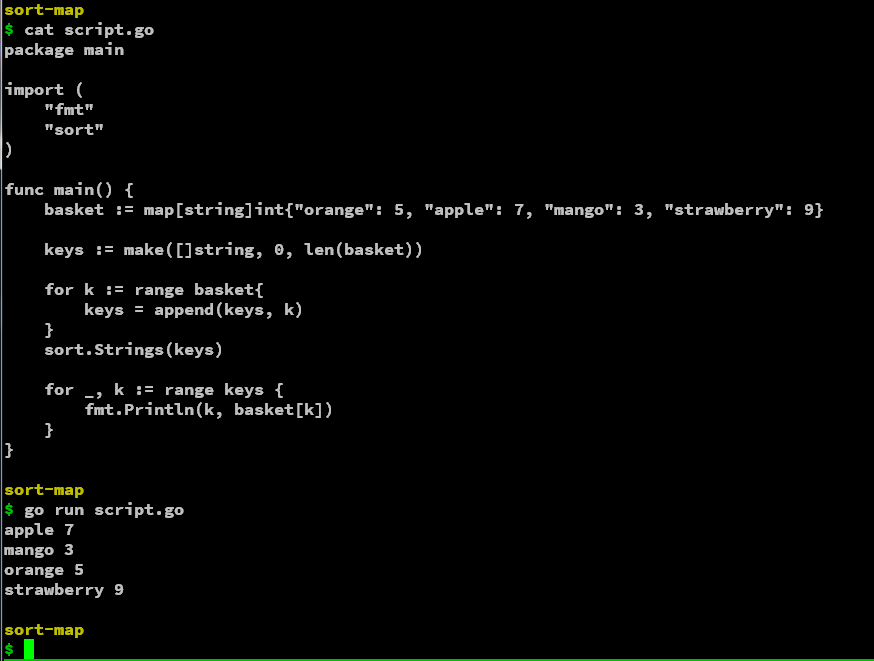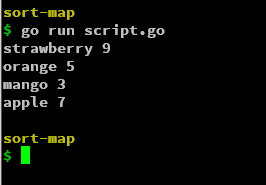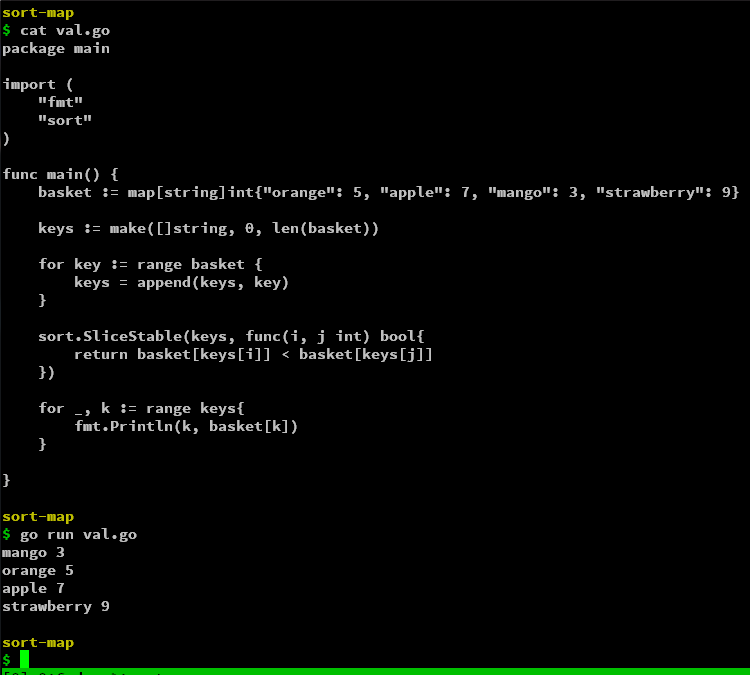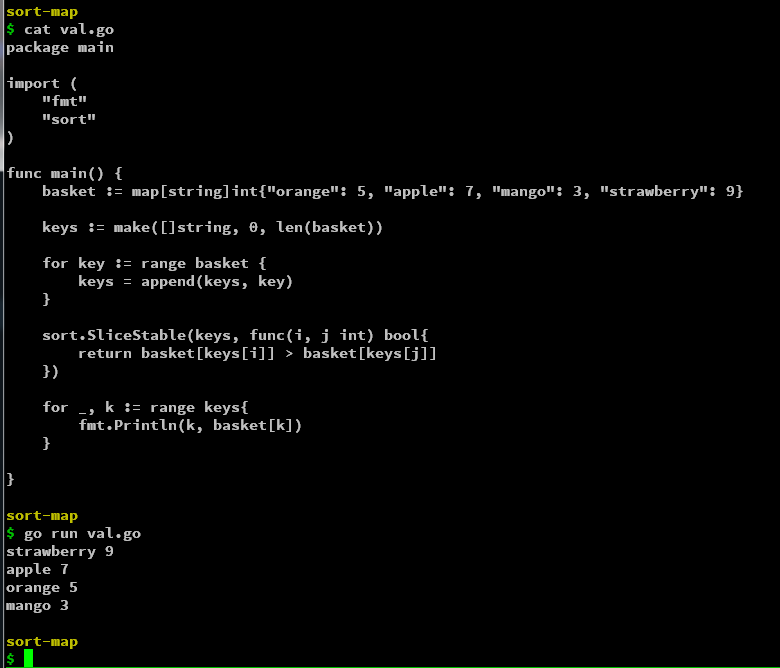Open in App
Not now

# How to Sort Golang Map By Keys or Values?

• Last Updated : 17 Oct, 2022

Let’s say we have a map and want to find a specific key-value pair but it can be located in any order, so to have the map in Golang in a particular order, we can sort the map by its keys or values. In this article, we will see how to sort a map in go lang by its keys or values.

### Sort By Keys

To sort a map by values, we need to first create a list of keys in that map(slices in Golang). By default Golang prints the map with sorted keys but while iterating over a map, it follows the order of the keys appearing as it is.

So, to sort the keys in a  map in Golang, we can create a slice of the keys and sort it and in turn sort the slice. Firstly we will iterate over the map and append all the keys in the slice. After we have all the keys we will use the sort.String function to sort the slice alphabetically. This will give a sorted slice/list of keys of the map. After that, we can simply iterate over this slice and access the value from the key in the map.

## Go

 `// Go program to sort the map by Keys``package` `main` `import` `(``    ``"fmt"``    ``"sort"``)` `func` `main() {``    ``basket := ``map``[``string``]int{``"orange"``: ``5``, ``"apple"``: ``7``,``                             ``"mango"``: ``3``, ``"strawberry"``: ``9``}` `    ``keys := make([]``string``, ``0``, len(basket))` `    ``for` `k := ``range` `basket{``        ``keys = append(keys, k)``    ``}``    ``sort.Strings(keys)` `    ``for` `_, k := ``range` `keys {``        ``fmt.Println(k, basket[k])``    ``}``}`

Output:We can see that we were able to iterate over the map by sorting the keys of a map. The keys can also be sorted in descending order using the Sort.Reverse function

We simply replace the sort.Strings(keys) with sort.Sort(sort.Reverse(sort.StringSlice(keys))) to get the slice in the descending alphabetical order.

## Go

 `// Go program to sort the map by Keys``package` `main` `import` `(``    ``"fmt"``    ``"sort"``)` `func` `main() {``    ``basket := ``map``[``string``]int{``"orange"``: ``5``, ``"apple"``: ``7``,``                             ``"mango"``: ``3``, ``"strawberry"``: ``9``}` `    ``keys := make([]``string``, ``0``, len(basket))` `    ``for` `k := ``range` `basket{``        ``keys = append(keys, k)``    ``}``    ``sort.Sort(sort.Reverse(sort.StringSlice(keys)))` `    ``for` `_, k := ``range` `keys {``        ``fmt.Println(k, basket[k])``    ``}``}`

Output:So the keys are obtained in reverse order of the original map.  You can further modify the sorting criteria with various functions in the sort module.

### Sort by Values

We can even iterate over the map by sorting the values, for that we need to use the sort.SliceStable function.

Firstly, similar to the sorting by key method, we have to obtain a slice of all the keys. Now we want to sort the keys according to the values, for doing that, we use the SliceStable function in the sort module. The slicestable function takes in the slice and we can provide less function. We can simply provide an anonymous/lambda fiction that checks for the comparison of the values of the provided slice. We compare the key at the ith index in the map so it becomes map[slice[i]], here keys is a slice of all the keys in the map and hence we access each key from the map. So, after this, we should have a sorted slice of keys as per the value of those keys.

We can test the output so far by the following script.

## Go

 `// Go program to sort the map by Values``package` `main` `import` `(``    ``"fmt"``    ``"sort"``)` `func` `main() {``    ``basket := ``map``[``string``]int{``"orange"``: ``5``, ``"apple"``: ``7``,``                             ``"mango"``: ``3``, ``"strawberry"``: ``9``}` `    ``keys := make([]``string``, ``0``, len(basket))` `    ``for` `key := ``range` `basket {``        ``keys = append(keys, key)``    ``}` `    ``fmt.Println(basket)``    ``fmt.Println(keys)` `    ``sort.SliceStable(keys, ``func``(i, j int) bool{``        ``return` `basket[keys[i]] < basket[keys[j]]``    ``})` `    ``fmt.Println(keys)``}`

Output:Here we can see the keys in the slice have been sorted by values in ascending order like [mango, orange, apple, strawberry] which have the value [3, 5, 7, 9] respectively. Further, we have to iterate over the keys splice and print the keys and values in the map.

## Go

 `// Go program to sort the map by Values``package` `main` `import` `(``    ``"fmt"``    ``"sort"``)` `func` `main() {``    ``basket := ``map``[``string``]int{``"orange"``: ``5``, ``"apple"``: ``7``,``                             ``"mango"``: ``3``, ``"strawberry"``: ``9``}` `    ``keys := make([]``string``, ``0``, len(basket))` `    ``for` `key := ``range` `basket {``        ``keys = append(keys, key)``    ``}``    ``sort.SliceStable(keys, ``func``(i, j int) bool{``        ``return` `basket[keys[i]] < basket[keys[j]]``    ``})` `    ``for` `_, k := ``range` `keys{``        ``fmt.Println(k, basket[k])``    ``}``}`

Output:So, we have iterated over the map in ascending order of its values. The keys here represent the splice so reiterate over it by the iterator k and a blank identifier as the index of the elements. So, we simply access the key with k and the value by using the map[k] in this case the map is the basket.

If we want to iterate over the map by sorting in descending order of the values, we simply have to modify the contents in the SliceStable less function.

## Go

 `package` `main` `import` `(``    ``"fmt"``    ``"sort"``)` `func` `main() {``    ``basket := ``map``[``string``]int{``"orange"``: ``5``, ``"apple"``: ``7``,``                             ``"mango"``: ``3``, ``"strawberry"``: ``9``}` `    ``keys := make([]``string``, ``0``, len(basket))` `    ``for` `key := ``range` `basket {``        ``keys = append(keys, key)``    ``}` `    ``sort.SliceStable(keys, ``func``(i, j int) bool{``        ``return` `basket[keys[i]] > basket[keys[j]]``    ``})` `    ``for` `_, k := ``range` `keys{``        ``fmt.Println(k, basket[k])``    ``}` `}`

Output:So by changing the less function return value to greater than the operator, we were able to sort the map in descending order. We were able to iterate over the map by sorting the keys in ascending/descending order. Similarly, we were able to iterate over the map by sorting the values of the keys in ascending and descending order. Thus, we were able to sort a map by its keys and values in Golang.

My Personal Notes arrow_drop_up